# initialCondition

Initial condition representation for linear time-invariant systems

## Description

An `initialCondition` object encapsulates the initial-condition information for a linear time-invariant (LTI) model. The object generalizes the numeric vector representation of the initial states of a state-space model so that the information applies to linear models of any form—transfer functions, polynomial models, or state-space models.

You can estimate and retrieve initial conditions when you identify a linear model using commands such as `tfest` or compare model response to measured input/output data using `compare`. The software estimates the initial condition value by minimizing the simulation or prediction error against the measured output data. You can then apply those initial conditions in a subsequent simulation, using commands such as `sim` or `predict`, to confirm model performance with respect to the same measurement data. Use the `initialCondition` command to create an `initialCondition` object from a state-space model specification or from any LTI model of a free response.

The `initialCondition` object can also be seen as a representation of the free response of a linear model. The simulation functions use this information to compute the model response in the following manner:

1. Compute the forced response of the model to the input signal. The forced response is the standard simulation output when there are no specified initial conditions.

2. Compute the impulse response of the model and scale the result to generate the free response of the model to the specified initial conditions.

3. Add the forced response and the free response together to form the total system response.

The figure illustrates this process.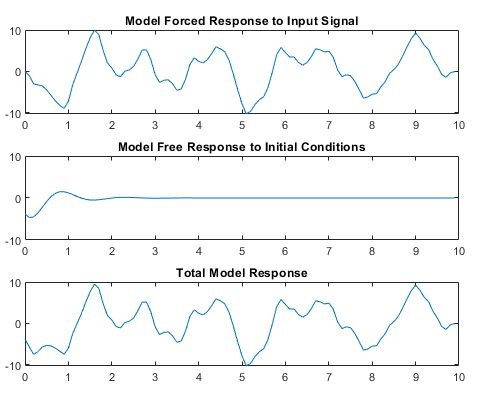For continuous systems (Ts = 0), the free response G(s) for the initial state vector x0 is

`$G\left(s\right)=C{\left(sI-A\right)}^{-1}{x}_{0}$`

Here, C is equivalent to the state-space measurement matrix C and A is equivalent to the state-space state matrix A.

For discrete systems (Ts >0), the free response G(z) is

`$G\left(z\right)=zC{\left(zI-A\right)}^{-1}{x}_{0}$`

The `initialCondition` object represents the free response in state-space form. The object is a structure with properties containing the state-space A and C matrices and the initial state vector x0. For `idtf` and `idpoly` models, using an `initialCondition` object is the only way to represent and use initial conditions for simulation. For `idss` models, you can use either an `initialCondition` object or a numeric initial state vector. When you obtain initial conditions `ic` for multiexperiment data, `ic` is an object array, with one `initialCondition` object for each experiment.

## Creation

You can obtain an `initialCondition` object in one of four ways.

• Model estimation — Specify that the estimation function return the estimated initial condition that corresponds to the estimation input/output data. For example, you can use the following command to obtain the estimated initial condition `ic` for a transfer function model `sys` that is estimated with input/output data `z`.

`[sys,ic] = tfest(z,2,1)`
For an example, see Obtain Estimated Initial Conditions.

• Model-to-data comparison using any input/output data — Specify that `compare` return the estimated initial condition that the function estimates internally to support the `fit` assessment. For example, you can use the following command to obtain the initial condition `ic` for the linear model `sys` when determining the fit against input/output data `z`. `yp` is the simulated or predicted model output.

`[yp,fit,ic] = compare(z,sys)`
For an example, see Obtain Initial Conditions for New Data.

• Direct construction — Use the `initialCondition` command to encapsulate the state-space form of a free-response model in an `initialCondition` object.

`ic = initialCondition(A,X0,C,Ts)`
For an example, see Construct initialCondition Object from State-Space Model.

• Free-response model conversion — Use the `initialCondition` command to convert an LTI free-response model into an `initialCondition` object.

`ic = initialCondition(G)`

For an example, see Convert Free-Response Model to initialCondition Object.

For information on functions you can use to extract information from or transform `initialCondition` objects, see Object Functions.

### Syntax

``ic = initialCondition(A,X0,C,Ts)``
``ic = initialCondition(G)``

### Description

example

````ic = initialCondition(A,X0,C,Ts)` creates an `initialCondition` object that represents the free response to an initial condition, expressed in state-space form, of an LTI model. $\begin{array}{l}dx=Ax\\ y=Cx\\ x\left(0\right)={x}_{0}\end{array}$`ic` stores this model in the form of properties. `A` and `C` correspond to a state-space realization of the model, `X0` to the initial state vector x0, and `Ts` to the sample time. You can use `ic` to specify initial conditions when simulating any type of LTI system.```

example

````ic = initialCondition(G)` creates an `initialCondition` object corresponding to a linear model `G` of the free response.```

### Input Arguments

expand all

Free-response model, specified as an LTI model with no inputs. In the continuous-time case, `G` must be strictly proper. In the discrete-time case, `G` must be biproper. For an example of using a free-response model to obtain an `initialCondition` object, see Convert Free-Response Model to initialCondition Object.

## Properties

expand all

A matrix of the state-space realization of the LTI free response, specified as an Nx-by-Nx numeric matrix, where Nx is the number of states. For an example of using this property, see Obtain Estimated Initial Conditions.

Initial states of the state-space realization of the LTI free response, specified as a numeric vector of length Nx. For an example of using this property, see Obtain Estimated Initial Conditions.

C matrix of the state-space realization of the LTI free response, specified as an Ny-by-Nx numeric matrix, where Ny is the number of outputs. For an example of using this property, see Obtain Estimated Initial Conditions.

Sample time of the LTI free response, specified as one of the following:

• Continuous-time model — 0

• Discrete-time model with a specified sampling time — Positive scalar representing the sampling period expressed in the unit specified by the `TimeUnit` property of the model

• Discrete-time model with unspecified sample time — –1

The sample time of an `initialCondition` object is the same as for the dynamic system model that the object corresponds to.

## Object Functions

Functions applicable to `initialCondition` objects are those that can return, use, or convert the objects.

Initial Condition (IC) RoleLTI Function TypeSyntax ExampleExample Links
Return estimated IC objectsAll estimation functions, `compare``[sys,ic] = tfest(data,2,1)`

Obtain Estimated Initial Conditions

Obtain Initial Conditions for Multiexperiment Data

Obtain Initial Conditions for New Data

Use IC objects for model responseOption sets for model response functions`opt = simOptions('InitialCondition',ic)`Apply Initial Conditions in Simulation
Convert IC objects into Dynamic System Models (DSMs)DSM object functions`g = idtf(ic)`Visualize Free Response to Initial Condition
Analyze models converted from IC objectsDSM analysis functions`y_g = impulse(g)`Visualize Free Response to Initial Condition

expand all

 `tfest` Estimate transfer function `procest` Estimate process model using time or frequency data `arx` Estimate parameters of ARX, ARIX, AR, or ARI model `armax` Estimate parameters of ARMAX, ARIMAX, ARMA, or ARIMA model using time-domain data `bj` Estimate Box-Jenkins polynomial model using time domain data `oe` Estimate output-error polynomial model using time-domain or frequency-domain data `polyest` Estimate polynomial model using time- or frequency-domain data `compare` Compare identified model output and measured output
 `sim` Simulate response of identified model `simOptions` Option set for `sim` `predict` Predict state and state estimation error covariance at next time step using extended or unscented Kalman filter, or particle filter `predictOptions` Option set for `predict` `pe` Prediction error for identified model `peOptions` Option set for `pe` `resid` Compute and test residuals `residOptions` Option set for `resid` `compare` Compare identified model output and measured output `compareOptions` Option set for `compare`
 `idss` State-space model with identifiable parameters `idpoly` Polynomial model with identifiable parameters `idtf` Transfer function model with identifiable parameters
 `impulse` Impulse response plot of dynamic system; impulse response data `freqresp` Frequency response over grid

## Examples

collapse all

Estimate a transfer function model and obtain estimated initial conditions.

```load iddata1ic.mat z1i plot(z1i)```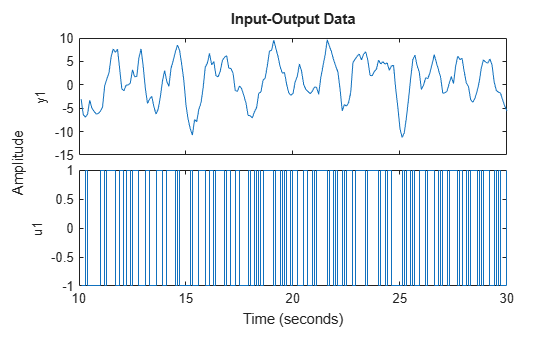The output data does not start at 0.

Estimate a second-order transfer function `sys_tf`. Specify that the function return the initial conditions `ic`.

`[sys_tf,ic] = tfest(z1i,2,1);`

Examine the contents of `ic`. `ic` includes, in state-space form, the free response model defined by matrices `A` and `C`, the initial state vector `X0`, and the sample time `Ts`.

`A = ic.A`
```A = 2×2 -2.9841 -5.5848 4.0000 0 ```
`C = ic.C`
```C = 1×2 0.2957 5.2441 ```
`x0 = ic.X0`
```x0 = 2×1 -0.9019 -0.6161 ```
`Ts = ic.Ts`
```Ts = 0 ```

`ic` is specific to the estimation data `z1i`. You can use `ic` to establish initial conditions when you simulate any LTI model using the input signal from `z1i` and compare the response with the `z1i` output signal.

Visualize the free response encapsulated in an `initialCondition` object by generating an impulse response.

Estimate a transfer function and return the initial condition `ic_tf`.

```load iddata1ic z1i [sys_tf,ic_tf] = tfest(z1i,2,1); ic_tf```
```ic_tf = initialCondition with properties: A: [2x2 double] X0: [2x1 double] C: [0.2957 5.2441] Ts: 0 ```

`ic_tf` contains the information necessary to compute the free response to an initial condition.

Convert `ic_tf` into an `idss` object that can be passed to the `impulse` function.

`ic_tfss = idss(ic_tf);`

Create a time vector `t` that spans the data set. Compute the impulse response.

```t = 0:0.1:9.9; t = t'; yimp = impulse(ic_tfss,t); plot(t,yimp) title('Free Response to Initial Condition')```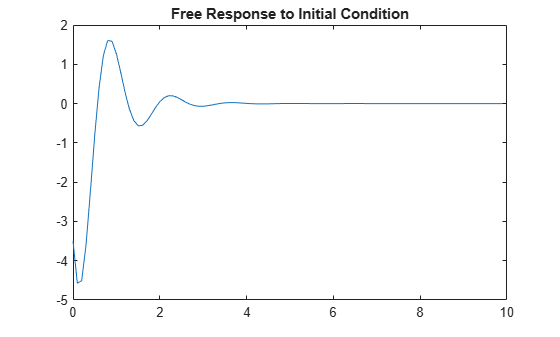The free response is a transient that lasts for about four seconds.

Load the data and estimate a second-order transfer function `sys`. Return initial conditions in `ic`.

```load iddata1ic z1i [sys,ic] = tfest(z1i,2,1);```

Simulate `sys` using the estimation data, but without incorporating the initial condition. Plot the simulated output with the measured output.

```y_no_ic = sim(sys,z1i); plot(y_no_ic,z1i) legend('Model Response','Measured Output')```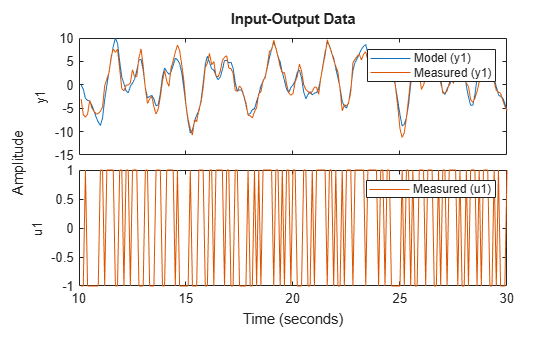The measured and simulated outputs do not agree at the beginning of the simulation.

Incorporate `ic` into the `simOptions` option set `opt`. Simulate and plot the model response using `opt`.

```opt = simOptions('InitialCondition',ic); y_ic = sim(sys,z1i,opt); plot(y_ic,z1i); legend('Model Response','Measured Output')```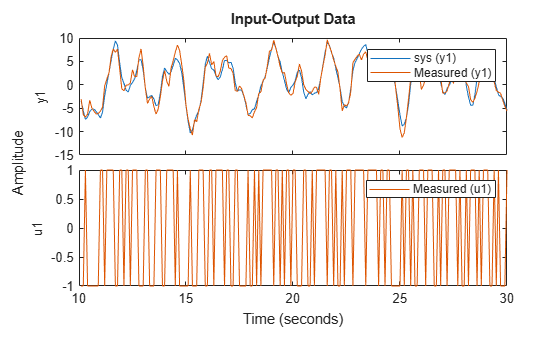The simulation combines the model response to the input signal with the free response to the initial condition. The measured and simulated outputs now have better agreement at the beginning of the simulation.

An estimated `initialCondition` object is specific to the data from which you estimated it. If you want to simulate your model with new data, such as validation data, you need to estimate a new initial condition for that data. To do so, use the `compare` command.

Load data and split it into estimation and validation data sets.

```load iddata1 z1 z1_est = z1(1:150); z1_val = z1(151:300); plot(z1_est,z1_val); legend('Estimation Data','Validation Data')```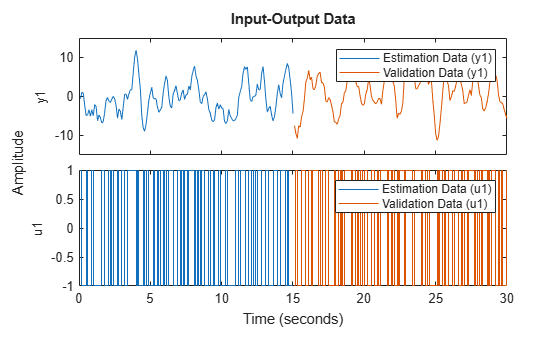Examine the start points of each output data set.

`e0 = z1_est.y(1)`
```e0 = -0.5872 ```
`v0 = z1_val.y(1)`
```v0 = -7.4390 ```

The two data sets have different starting conditions.

Estimate a second-order transfer function model using `z1_est`. Return the estimated initial conditions in `ic_est`. Display the `X0` property of `ic_est`. This property represents the estimated initial state vector that the free-response model defined by `ic_est.A` and `ic_est.C` responds to.

```[sys,ic_est] = tfest(z1_est,2,1); ic_est.X0```
```ans = 2×1 -0.4082 0.0095 ```

You can use `ic_est` if you want to simulate `sys` using `z1_est`. Alternatively, you can use `compare`, which estimates the initial condition independently. Use `compare` twice, once to plot the data and once to return the results. Display the initial state vector `ic_estc.X0` that `compare` estimates.

`compare(z1_est,sys)`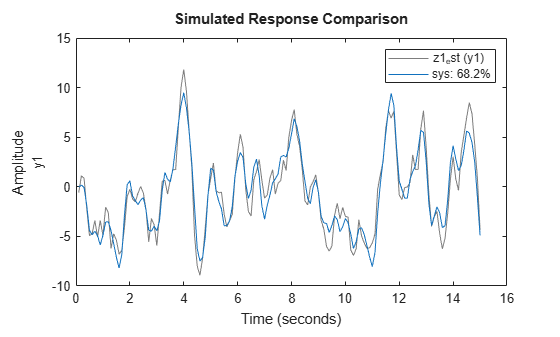```[yce,fit,ic_estc] = compare(z1_est,sys); ic_estc.X0```
```ans = 2×1 -0.4082 0.0095 ```

The initial state vector `ic_estc.X0` is identical to `ic_est`.

Now evaluate the model with the validation data set. Estimate the initial conditions with the validation data.

`compare(z1_val,sys)`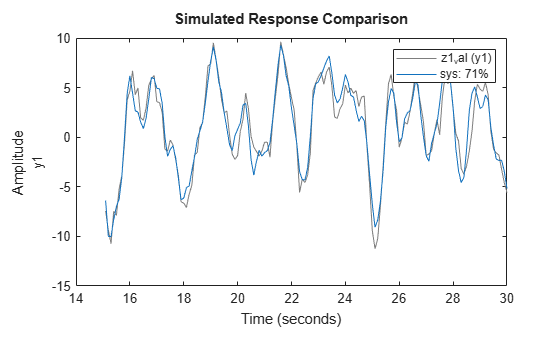```[ycv,fit,ic_valc] = compare(z1_val,sys); ic_valc.X0```
```ans = 2×1 -1.7536 -0.9547 ```

You can use `ic_val` when you simulate `sys` with the `z1_val` input signal and compare the model response to the `z1_val` output signal.

Estimate an `initialCondition` object array using multiexperiment data.

Load data from two experiments. Merge the two data sets into one multiexperiment data set.

```load iddata1 z1 load iddata2 z2 z12 = merge(z1,z2)```
```z12 = Time domain data set containing 2 experiments. Experiment Samples Sample Time Exp1 300 0.1 Exp2 400 0.1 Outputs Unit (if specified) y1 Inputs Unit (if specified) u1 ```
`plot(z12)`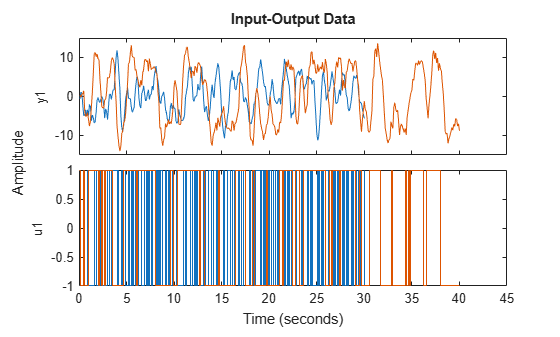Estimate the second-order transfer function `sys` and return the initial conditions in `ic`.

```np = 2; nz = 1; [sys,ic] = tfest(z12,np,nz); ic```
```ic=1×2 object 1x2 initialCondition array with properties: A X0 C Ts ```

`ic` is an object array. Display the contents of each object.

`ic(1,1)`
```ans = initialCondition with properties: A: [2x2 double] X0: [2x1 double] C: [-0.7814 5.2530] Ts: 0 ```
`ic(1,2)`
```ans = initialCondition with properties: A: [2x2 double] X0: [2x1 double] C: [-0.7814 5.2530] Ts: 0 ```

Compare the `A`, `X0`, and `C` properties for each object.

`A1 = ic(1,1).A`
```A1 = 2×2 -3.4824 -5.5785 4.0000 0 ```
`A2 = ic(1,2).A`
```A2 = 2×2 -3.4824 -5.5785 4.0000 0 ```
`C1 = ic(1,1).C`
```C1 = 1×2 -0.7814 5.2530 ```
`C2 = ic(1,2).C`
```C2 = 1×2 -0.7814 5.2530 ```
`X01 =ic(1,1).X0`
```X01 = 2×1 -0.6528 -0.0067 ```
`X02 =ic(1,2).X0`
```X02 = 2×1 0.3076 -0.0715 ```

The `A` and `C` matrices are identical. These matrices represent the state-space form of `sys`. The `X0` vectors are different. This difference results from the different initial conditions for the two experiments.

Estimate a state-space model and return the initial states. From the model and the initial state vector, construct an `initialCondition` object that can be used with any linear model.

```load iddata1ic z1i plot(z1i)```Estimate a state-space model and obtain the estimated initial conditions.

First, set the `'InitialState'` name-value pair argument in `ssestOptions` to `'estimate'`, which overrides the default setting of `'auto'`. The `'estimate'` setting always estimates the initial states. The `'auto'` setting uses the `'zero'` setting if the effect of the initial states on the overall model estimation error is relatively small, and can therefore result in an initial-state vector containing only zeros.

```opt = ssestOptions; opt = ssestOptions('InitialState','estimate');```

Estimate a second-order state-space model `sys_ss`. Specify the output argument `x0` to return the initial state vector. Specify the input argument `opt` to use your '`InitialState'` setting. After estimating, examine `x0`.

```[sys_ss,x0] = ssest(z1i,2,opt); x0```
```x0 = 2×1 0.0631 0.0329 ```

`x0` is a nonzero initial state vector.

Simulate the model using `x0` and compare the output with the original output data.

To use `x0` as the initial condition, specify the `'InitialCondition'` name-value pair argument in `simOptions` as `x0`.

```opt = simOptions; opt = simOptions('InitialCondition',x0);```

Simulate the model using `opt` and store the response in `xss`.

`xss = sim(sys_ss,z1i,opt);`

Plot the model response with the original output data.

```t = 0:0.1:19.9; plot(t',[xss.y z1i.y]) legend('ss model','output data') title('Simulated State-Space Model Using Estimated Initial States')```The simulation starts at a point close to the starting point of the data.

With the `A` and `C` matrices, `x0`, and the sample time `Ts` from `sys_ss`, construct an `initialCondition` object `ic` that you can use with a transfer function model.

```A = sys_ss.A; C = sys_ss.C; Ts = sys_ss.Ts; ic = initialCondition(A,x0,C,Ts)```
```ic = initialCondition with properties: A: [2x2 double] X0: [2x1 double] C: [-61.3674 13.4811] Ts: 0 ```

Estimate a transfer function model and simulate the model using `ic` as the initial condition. Store the response in `xtf`.

```sys_tf = tfest(z1i,2,1); opt = simOptions('InitialCondition',ic); xtf = sim(sys_tf,z1i,opt);```

Plot the model responses `xss` and `xtf` together.

```plot(t',[xss.y xtf.y]) legend('ss model','tf model') title('Simulated SS and TF Models with Equivalent Initial Conditions')```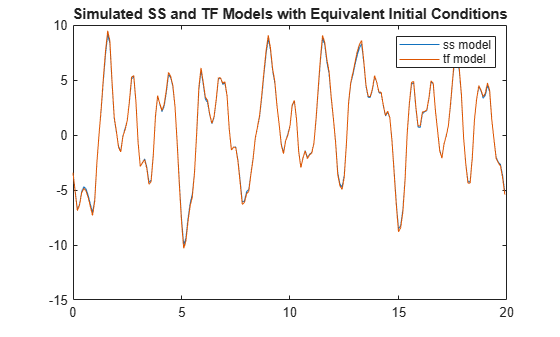The models track each other closely throughout the simulation.

Obtain the initial conditions when estimating a transfer function model. Convert the `initialCondition` into a free-response model, and the free-response model back into an `initialCondition` object.

Load the data and estimate a transfer function model `sys`. Obtain the estimated initial conditions `ic`.

```load iddata1ic.mat z1i [sys,ic] = tfest(z1i,2,1);```

Convert `ic` into the `idtf` free-response model `g`.

`g = idtf(ic);`

Plot the impulse response of `g`.

```impulse(g) title('Impulse Response of g')```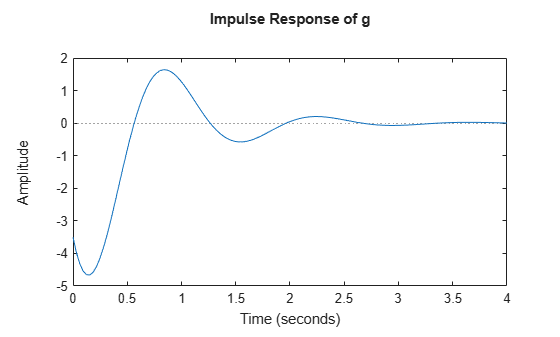Convert `g` back into the `initialCondition` object `ic1`.

`ic1 = initialCondition(g);`

Plot the impulse response of `ic1` by converting `ic1` into an `idss` model.

```impulse(idss(ic1)) title('Impulse Response of ic1 in idss form')```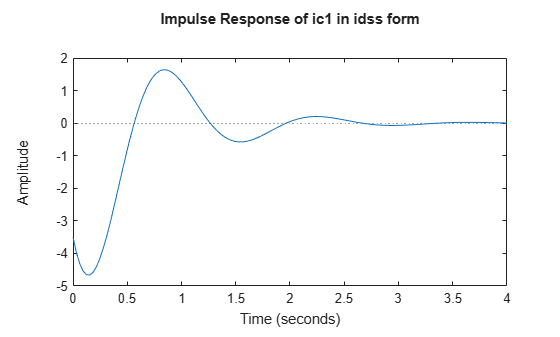The impulse responses appear identical.

Compare `ic` and `ic1`.

`ic.A`
```ans = 2×2 -2.9841 -5.5848 4.0000 0 ```
`ic1.A`
```ans = 2×2 -2.9841 -5.5848 4.0000 0 ```
`ic.X0`
```ans = 2×1 -0.9019 -0.6161 ```
`ic1.X0`
```ans = 2×1 4 0 ```
`ic.C`
```ans = 1×2 0.2957 5.2441 ```
`ic1.C`
```ans = 1×2 -0.8745 -1.7215 ```

The `A` matrices of `ic` and `ic1` are identical. The `C` matrix and the `X0` vector are different. There are infinitely many state-space representations possible for a given linear model. The two objects are equivalent, as illustrated by the impulse responses.